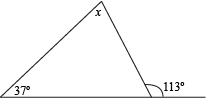# Find the measure of x.76°

Explanation

We can use the Angle Sum Theorem to find x, but for that to work, we have to find the other angles of the triangle. Luckily, we can do that.

One of the angles is already marked as 37°. One down; two to go.

We aren't given any other direct measurements, but another interior angle in the triangle has a supplementary angle of 113°. Since we know supplementary angles add up to 180°, the other angle has a measure of 180° – 113° = 67°.

All angles in a triangle add up to 180° (thanks, Angle Sum Theorem), so we can add the angles up to find x.

37 + 67 + x = 180
x = 76